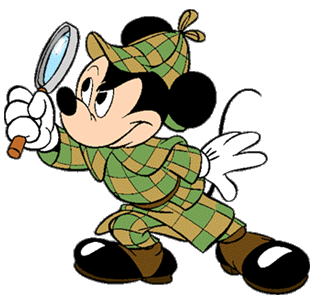SAT Math XIX

Algebra Level 1If $y=3^x$ and $x$ and $y$ are both integers, which of the following is equivalent to $9^x+3^{x+1}$?

×